Ex 5.4 (Optional)

Chapter 5 Class 10 Arithmetic Progressions
Serial order wise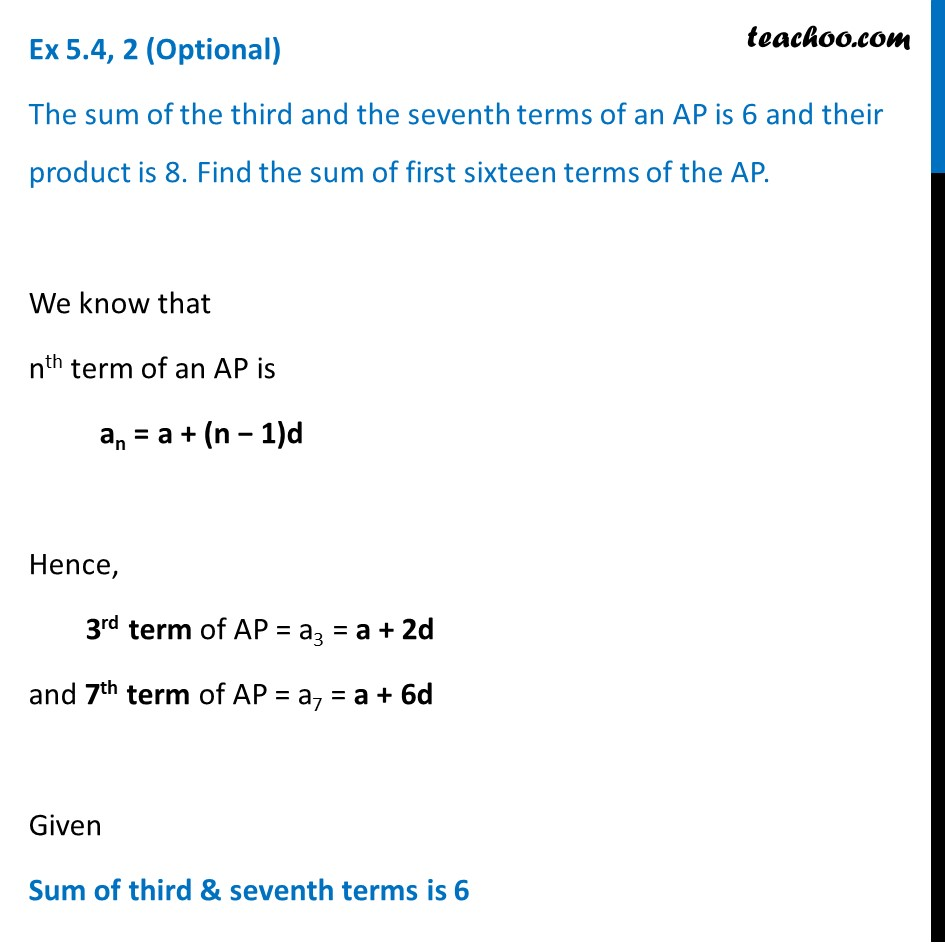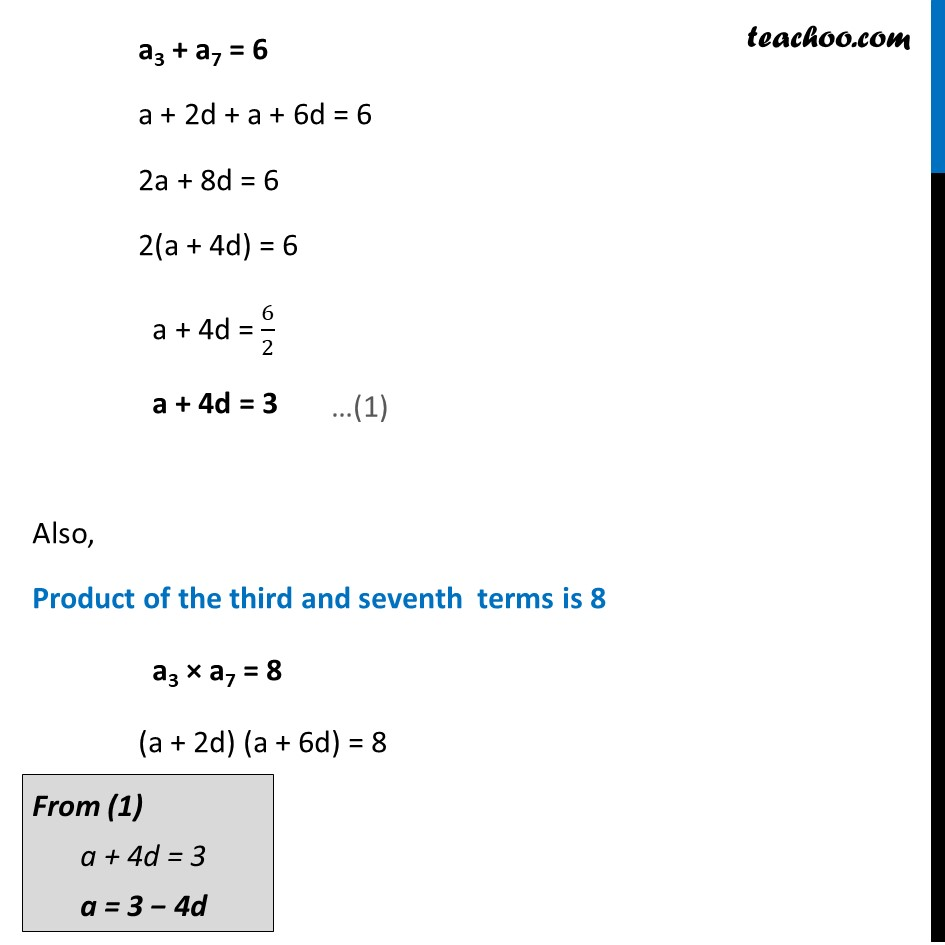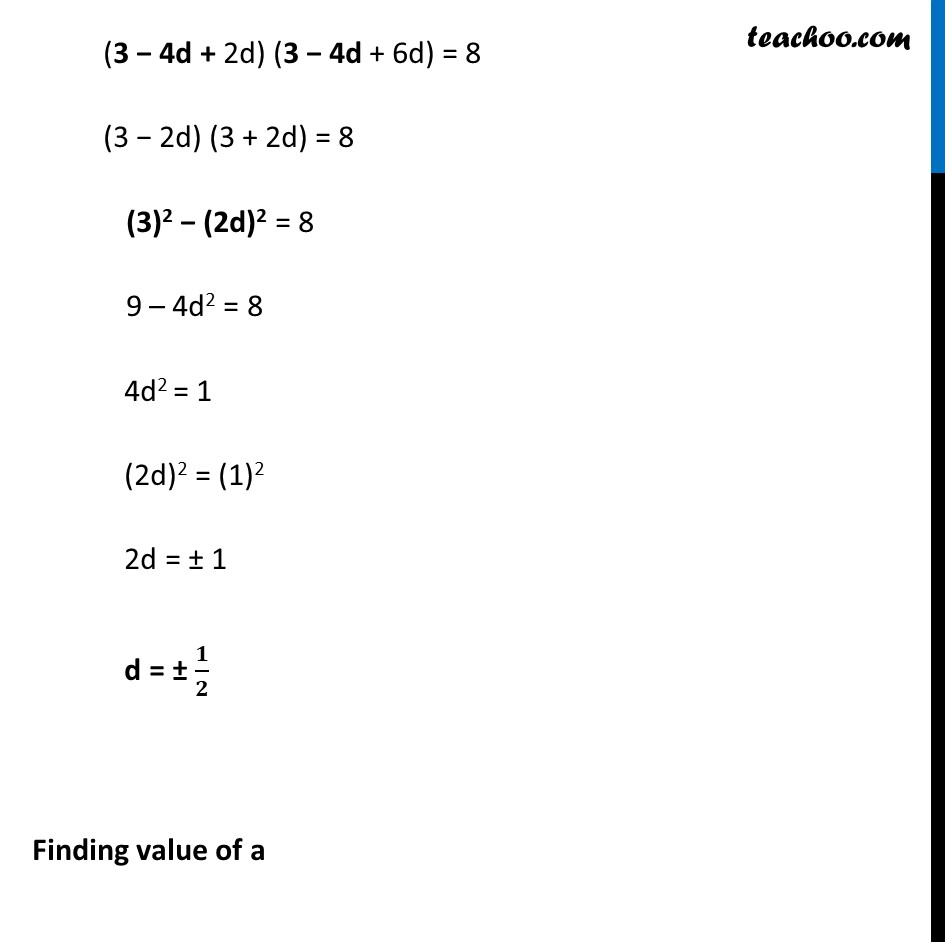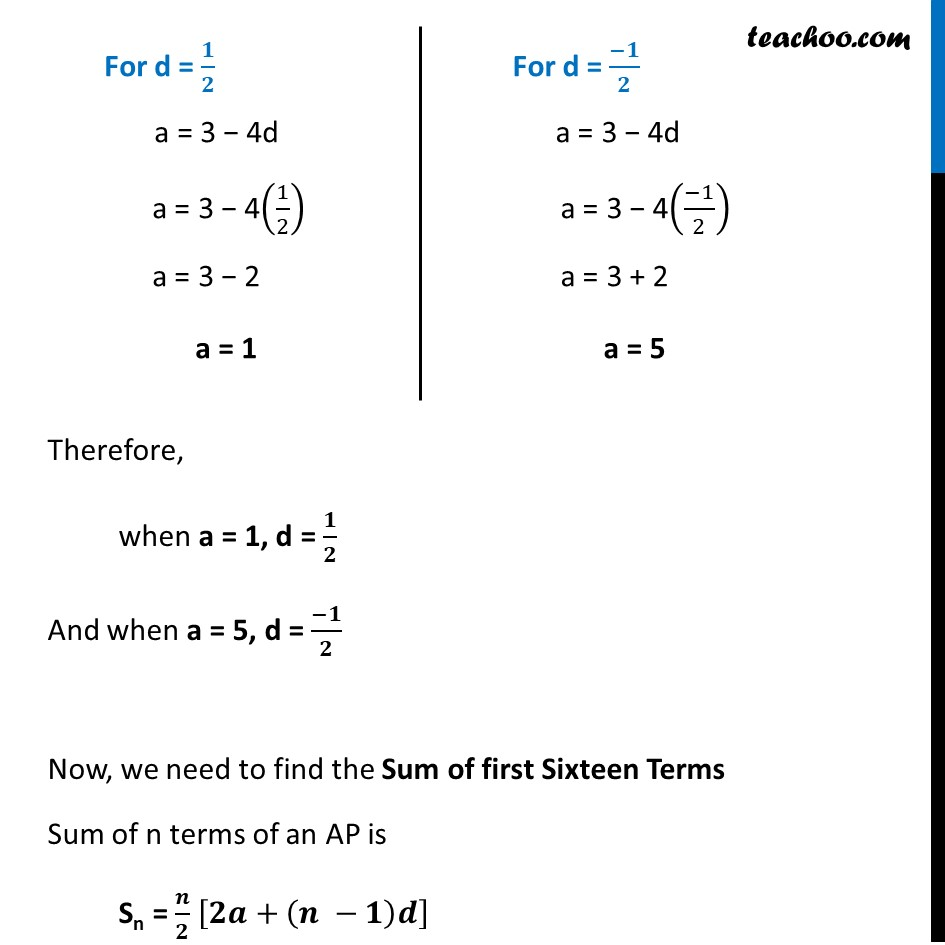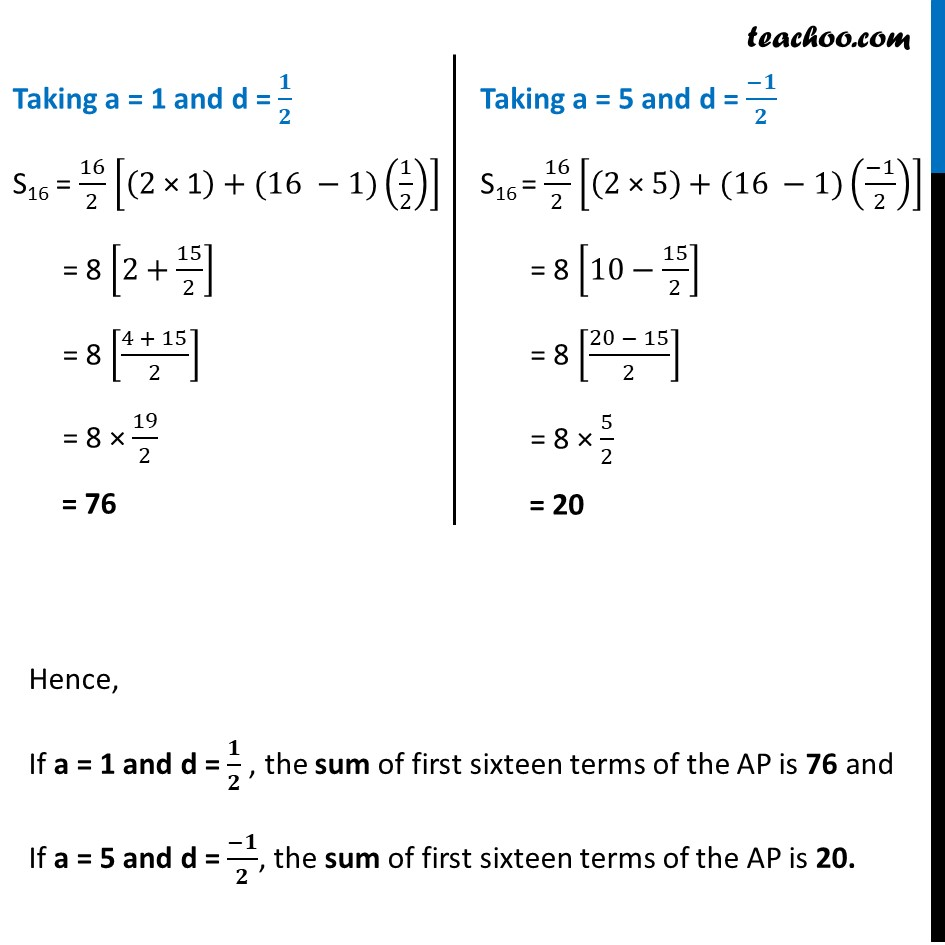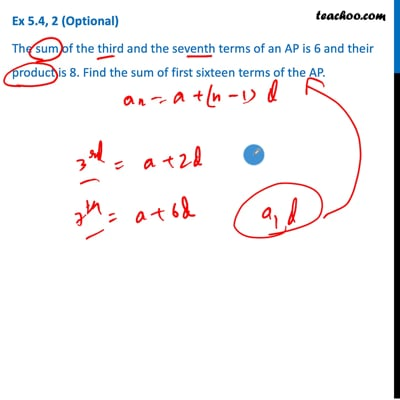This video is only available for Teachoo black users

Maths Crash Course - Live lectures + all videos + Real time Doubt solving!

### Transcript

Ex 5.4, 2 (Optional) The sum of the third and the seventh terms of an AP is 6 and their product is 8. Find the sum of first sixteen terms of the AP. We know that nth term of an AP is an = a + (n − 1)d Hence, 3rd term of AP = a3 = a + 2d and 7th term of AP = a7 = a + 6d Given Sum of third & seventh terms is 6 a3 + a7 = 6 a + 2d + a + 6d = 6 2a + 8d = 6 2(a + 4d) = 6 a + 4d = 6/2 a + 4d = 3 a3 + a7 = 6 a + 2d + a + 6d = 6 2a + 8d = 6 2(a + 4d) = 6 a + 4d = 6/2 a + 4d = 3 Also, Product of the third and seventh terms is 8 a3 × a7 = 8 (a + 2d) (a + 6d) = 8 From (1) a + 4d = 3 a = 3 − 4d (3 − 4d + 2d) (3 − 4d + 6d) = 8 (3 − 2d) (3 + 2d) = 8 (3)2 − (2d)2 = 8 9 – 4d2 = 8 4d2 = 1 (2d)2 = (1)2 2d = ± 1 d = ± 𝟏/𝟐 Finding value of a For d = 𝟏/𝟐 a = 3 − 4d a = 3 − 4(1/2) a = 3 − 2 a = 1 For d = (−𝟏)/𝟐 a = 3 − 4d a = 3 − 4((−1)/2) a = 3 + 2 a = 5 For d = (−𝟏)/𝟐 a = 3 − 4d a = 3 − 4((−1)/2) a = 3 + 2 a = 5 Therefore, when a = 1, d = 𝟏/𝟐 And when a = 5, d = (−𝟏)/𝟐 Now, we need to find the Sum of first Sixteen Terms Sum of n terms of an AP is Sn = 𝒏/𝟐 [𝟐𝒂+(𝒏 −𝟏)𝒅] Taking a = 1 and d = 𝟏/𝟐 S16 = 16/2 [(2" × 1" )+(16 −1)(1/2)] = 8 [2+15/2] = 8 [(4 + 15)/2] = 8 × 19/2 = 76 Taking a = 5 and d = (−𝟏)/𝟐 S16 = 16/2 [(2" × " 5)+(16 −1)((−1)/2)] = 8 [10−15/2] = 8 [(20 − 15)/2] = 8 × 5/2 = 20 Hence, If a = 1 and d = 𝟏/𝟐 , the sum of first sixteen terms of the AP is 76 and If a = 5 and d = (−𝟏)/𝟐, the sum of first sixteen terms of the AP is 20.